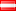# DSP## Digital Signal Processing

In order to use an analog signal on a computer it must be digitized with an analog-to-digital converter. Sampling is usually carried out in two stages, discretization and quantization. In the discretization stage, the space of signals is partitioned into equivalence classes and quantization is carried out by replacing the signal with representative signal of the corresponding equivalence class. In the quantization stage the representative signal values are approximated by values from a finite set. The Nyquist–Shannon sampling theorem states that a signal can be exactly reconstructed from its samples if the sampling frequency is greater than twice the highest frequency of the signal; but requires an infinite number of samples . In practice, the sampling frequency is often significantly more than twice that required by the signal's limited bandwidth. A digital-to-analog converter is used to convert the digital signal back to analog. The use of a digital computer is a key ingredient in digital control systems.

## Courses

 Updated at: to the top